# ISEE Middle Level Math : How to find the perimeter of the rectangle

## Example Questions

1 2 4 Next →

### Example Question #89 : Rectangles

Find the perimeter of a rectangle with a length of 36cm and a width that is a third of the length.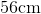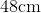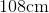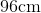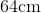Explanation:

To find the perimeter of a rectangle, we will use the following formula: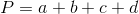Where a, b, c, and d are the lengths of the sides of the rectangle.

Now, we know the length of the rectangle is 36cm.  Because it is a rectangle, the opposite side is also 36cm.  We also know the width is a third of the length.  Therefore, the width is 12cm.  Because it is a rectangle, the opposite side is also 12cm.

Knowing this, we can substitute into the formula.  We get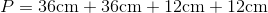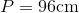### Example Question #90 : Rectangles

Find the perimeter of a rectangle with a width of 6in and a length that is four times the width.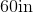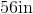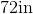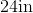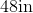Explanation:

To find the perimeter of a rectangle, we will use the following formula:Where a, b, c, and d are the lengths of the sides of the rectangle.

Now, we know the width of the rectangle is 6in.  Because it is a rectangle, the opposite side is also 6in.

We know the length is four times the width.  Therefore, the length is 24in.  Because it is a rectangle, the opposite side is also 24in.

Knowing this, we can substitute into the formula.  We get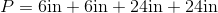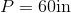### Example Question #31 : How To Find The Perimeter Of The Rectangle

Find the perimeter of a rectangle with a width of 2cm and a length that is six times the width.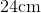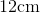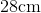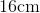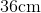Explanation:

To find the perimeter of a rectangle, we will use the following formula:Where a, b, c, and d are the lengths of the sides of the rectangle.

Now, we know the width of the rectangle is 2cm.  Because it is a rectangle, the opposite side is also 2cm.

We know the length is six times the width.  Therefore, the length is 12cm.  Because it is a rectangle, the opposite side is also 12cm.

Knowing this, we can substitute into the formula.  We get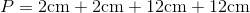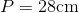### Example Question #251 : Geometry

Use the following rectangle to answer the question: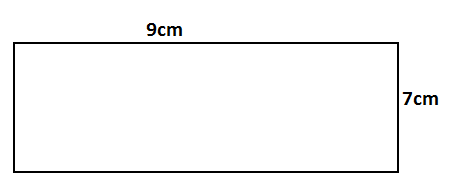Find the perimeter.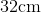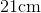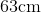Explanation:

To find the perimeter of a rectangle, we will use the following formula:Where a, b, c, and d are the lengths of the sides of the rectangle.

Now, given the rectangle,we can see the length is 9cm.  Because it is a rectangle, the opposite side is also 9cm.

We can see the width is 7cm.  Because it is a rectangle, the opposite side is also 7cm.

Knowing this, we can substitute into the formula.  We get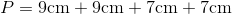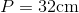### Example Question #252 : Geometry

Find the perimeter of a rectangle with a width of 6in and a length that is three times the width.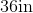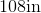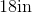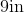Explanation:

To find the perimeter of a rectangle, we will use the following formula:Where a, b, c, and d are the lengths of the sides of the rectangle.

Now, we know the width of the rectangle is 6in.  Because it is a rectangle, the opposite side is also 6in.

We know the length is three times the width.  Therefore, the length is 18in.  Because it is a rectangle, the opposite side is also 18in.

So, we can substitute.  We get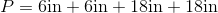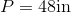### Example Question #253 : Geometry

Find the perimeter of a rectangle with a width of 4cm and a length that is three times the width.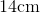Explanation:

To find the perimeter of a rectangle, we will use the following formula:Where a, b, c, and d are the lengths of the sides of the rectangle.

Now, we know the width of the rectangle is 4cm.  Because it is a rectangle, the opposite side is also 4cm.

We know the length is three times the width.  Therefore, the length is 12cm.  Because it is a rectangle, the opposite side is also 12cm.

So, we can substitute.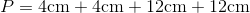### Example Question #254 : Geometry

Find the perimeter of a rectangle with a width of 6in and a length that is two times the width.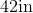Explanation:

To find the perimeter of a rectangle, we will use the following formula:Where a, b, c, and d are the lengths of the sides of the rectangle.

Now, we know the width of the rectangle is 6in.  Because it is a rectangle, the opposite side is also 6in.

We know the length is two times the width.  Therefore, the length is 12in.  Because it is a rectangle, the opposite side is also 12in.

So, we get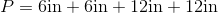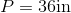### Example Question #255 : Geometry

Find the perimeter of a rectangle with a length of 16cm and a width that is a quarter of the length.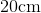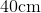Explanation:

To find the perimeter of a rectangle, we will use the following formula:Where a, b, c, and d are the lengths of the sides of the rectangle.

Now, we know the length of the rectangle is 16cm.  Because it is a rectangle, the opposite side is also 16cm.  We also know the width of the rectangle is a quarter of the length.  To find the width, we will divide 16 by 4.  Therefore, the width is 4cm.  Because it is a rectangle, the opposite side is also 4cm.

Knowing this, we can substitute into the formula.  We get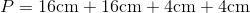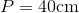### Example Question #256 : Geometry

Find the perimeter of a rectangle with an area of, and a base length of.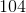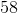Explanation:

This problem requires finding the height of the rectangle first.

Write the formula for the area of the rectangle, and substitute the known values.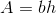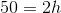Divide by two on both sides to obtain the height.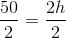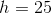The perimeter of a rectangle includes 2 bases and 2 heights.

The formula for the perimeter is: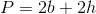Substitute the values to find the perimeter.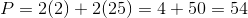The perimeter is:1 2 4 Next →